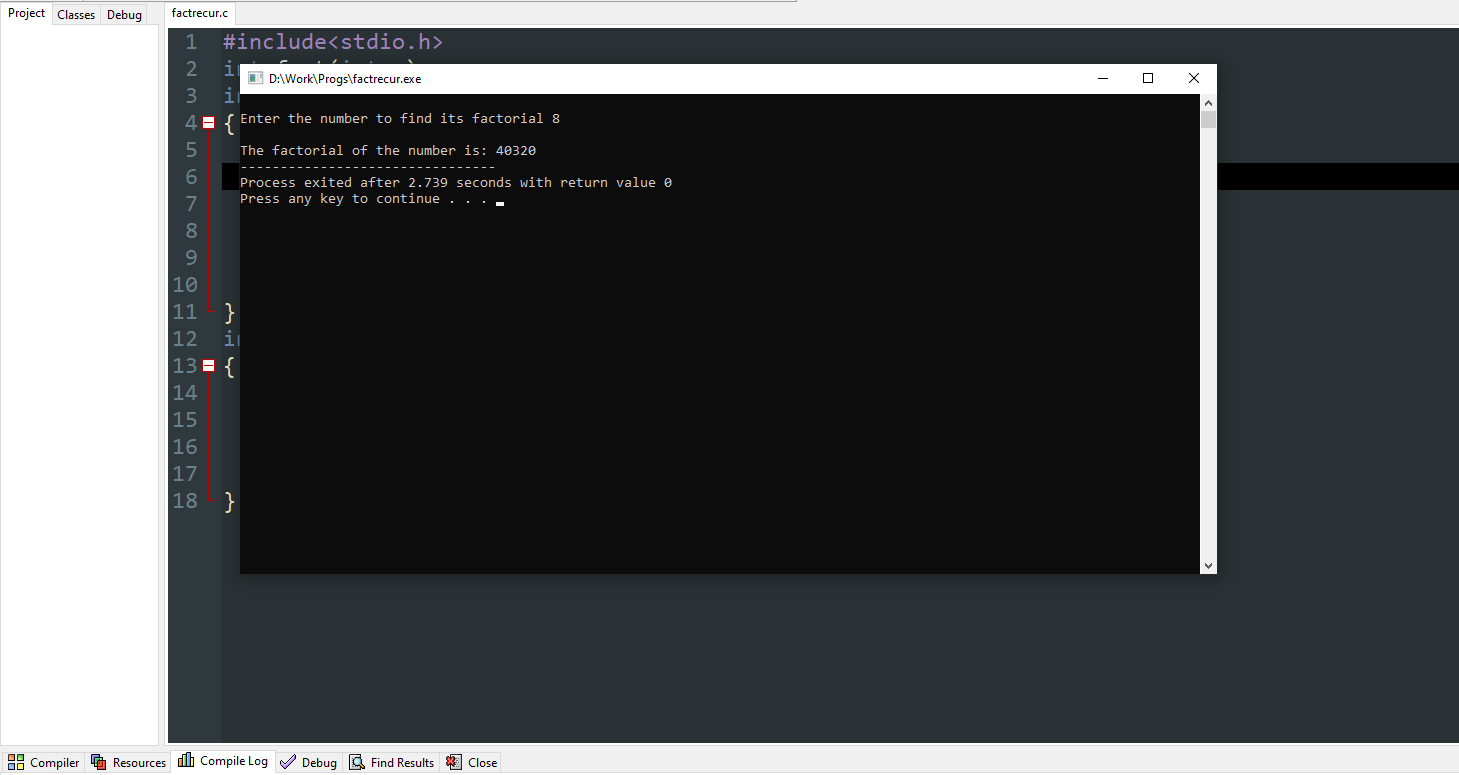# What is recursion in C?

Here we are going to be discuss about, What’s is recursion in C?

Recursion is a function which calls itself and keeps on repeating until the condition is satisfied for the termination of the process.

## Syntax Of Recursion In C:

```datatype functionname()
{
functionname ();
}
datatype main()
{
functionname ();
}```

### Summary:

Here in the above example, it shows how recursion actually works. The function demo calls itself again and again until the termination statement is satisfied as provided by the user. This is a simple logic which is mostly used in programs to find the factorial of Fibonacci series (most common question).

Let us take some program examples to get the recursion cleared.

## Program to find factorial through recursion:

```#include<stdio.h>
int fact(int n);
int main()
{
int n,f;
printf("\nEnter the number to find its factorial ");
scanf("%d",&n);
f=fact(n);
printf("\nThe factorial of the number is: %d",f);
return 0;
}
int fact(int n)
{
if(n==0)
return 1;
else
return (n*fact(n-1));
}```

### Explanation:

Step-1: We take a variable n which is declared as the number, whose factorial is calculated and a variable f where the return value of the function calculating recursion is received.

Step-2: Then we call the function declared as fact in f.

Step-3: In the function fact, it checks if the variable is zero or not, if the variable is zero then it returns 1 where the factorial of 0 is 1.

Step-4: In the else case it returns the recursive case: n*fact(n-1)

Step-5: where n is multiplied by itself-1 in every case of recursion, where the value of n gets decremented by 1 in every case till it reaches 0.

Step-6: In the end the value received in f is printed to receive the factorial of the number.

### Output:

``````We have taken input of the number as 8.
Where fact of 8 is:
1*2*3*4*5*6*7*8=40320
``````This is how recursion is received in C which is explained through the program to find the factorial of a number.##### Soham Malakar

Hello my name is Soham Malakar am currently pursuing BCA

Coding is one of my favorite hobbies.
I love to code and an enthusiast to learn different types of programming languages.
I love to get intoxicated in the world of technology and video games.
A quick learner possessing better results.

Articles: 40